# Detailed Data Analysis & Simple CatBoost - 0.640 on LB

Description of features and the entire dataset, selection of categorical features by logic, CatBoost

I tried to make detailed graphs of the features and their dependencies on the diagnosis. I also made basic summaries for the entire dataset and trained the model. The analysis of the dataset corresponds to the organizers pdf. So, you can easily find the description of the desired feature in pdf. LB scores is 0.640 and 0.447.

# Setup AIcrowd Utilities 🛠¶

In [ ]:
!pip install -q -U aicrowd-cli

In [ ]:
%load_ext aicrowd.magic


# AIcrowd Runtime Configuration 🧷¶

In [ ]:
import os

# Please use the absolute for the location of the dataset.
# Or you can use relative path with os.getcwd() + "test_data/validation.csv"
AICROWD_TRAIN_DATASET_PATH = os.getenv("TRAIN_DATASET_PATH", "/ds_shared_drive/train.csv")
AICROWD_DATASET_PATH = os.getenv("DATASET_PATH", "/ds_shared_drive/validation.csv")
AICROWD_PREDICTIONS_PATH = os.getenv("PREDICTIONS_PATH", "predictions.csv")
AICROWD_ASSETS_DIR = "assets"


# Install packages 🗃¶

In [ ]:
!pip install numpy pandas catboost sklearn

Requirement already satisfied: numpy in ./conda/lib/python3.8/site-packages (1.20.2)
Requirement already satisfied: pandas in ./conda/lib/python3.8/site-packages (1.2.4)
Requirement already satisfied: catboost in ./conda/lib/python3.8/site-packages (0.25.1)
Requirement already satisfied: sklearn in ./conda/lib/python3.8/site-packages (0.0)
Requirement already satisfied: python-dateutil>=2.7.3 in ./conda/lib/python3.8/site-packages (from pandas) (2.8.1)
Requirement already satisfied: pytz>=2017.3 in ./conda/lib/python3.8/site-packages (from pandas) (2021.1)
Requirement already satisfied: scipy in ./conda/lib/python3.8/site-packages (from catboost) (1.6.3)
Requirement already satisfied: matplotlib in ./conda/lib/python3.8/site-packages (from catboost) (3.4.1)
Requirement already satisfied: graphviz in ./conda/lib/python3.8/site-packages (from catboost) (0.16)
Requirement already satisfied: six in ./conda/lib/python3.8/site-packages (from catboost) (1.15.0)
Requirement already satisfied: plotly in ./conda/lib/python3.8/site-packages (from catboost) (4.14.3)
Requirement already satisfied: scikit-learn in ./conda/lib/python3.8/site-packages (from sklearn) (0.24.2)
Requirement already satisfied: pyparsing>=2.2.1 in ./conda/lib/python3.8/site-packages (from matplotlib->catboost) (2.4.7)
Requirement already satisfied: pillow>=6.2.0 in ./conda/lib/python3.8/site-packages (from matplotlib->catboost) (8.2.0)
Requirement already satisfied: cycler>=0.10 in ./conda/lib/python3.8/site-packages (from matplotlib->catboost) (0.10.0)
Requirement already satisfied: kiwisolver>=1.0.1 in ./conda/lib/python3.8/site-packages (from matplotlib->catboost) (1.3.1)
Requirement already satisfied: retrying>=1.3.3 in ./conda/lib/python3.8/site-packages (from plotly->catboost) (1.3.3)
Requirement already satisfied: joblib>=0.11 in ./conda/lib/python3.8/site-packages (from scikit-learn->sklearn) (1.0.1)


# Define preprocessing code¶

## Import common packages¶

In [ ]:
import numpy as np
import os
import random
import pandas as pd
import matplotlib.pyplot as plt
import seaborn as sns
from sklearn.model_selection import train_test_split
from catboost import CatBoostClassifier


# Training phase¶

In [ ]:
train_data = pd.read_csv(AICROWD_TRAIN_DATASET_PATH)

Out[ ]:
row_id number_of_digits missing_digit_1 missing_digit_2 missing_digit_3 missing_digit_4 missing_digit_5 missing_digit_6 missing_digit_7 missing_digit_8 ... bottom_area_perc left_area_perc right_area_perc hor_count vert_count eleven_ten_error other_error time_diff centre_dot_detect diagnosis
0 S0CIXBKIUEOUBNURP 12.0 0.0 0.0 0.0 0.0 0.0 0.0 0.0 0.0 ... 0.526170 0.524975 0.474667 0 0 0 1 -105.0 0.0 normal
1 IW1Z4Z3H720OPW8LL 12.0 0.0 0.0 0.0 0.0 0.0 0.0 0.0 0.0 ... 0.000810 0.516212 0.483330 0 1 0 1 NaN NaN normal
2 PVUGU14JRSU44ZADT 12.0 0.0 0.0 0.0 0.0 0.0 0.0 0.0 0.0 ... 0.488109 0.550606 0.449042 0 0 0 0 0.0 0.0 normal
3 RW5UTGMB9H67LWJHX 7.0 0.0 0.0 0.0 1.0 0.0 0.0 1.0 1.0 ... NaN NaN NaN 1 0 0 1 NaN NaN normal
4 W0IM2V6F6UP5LYS3E 12.0 0.0 0.0 0.0 0.0 0.0 0.0 0.0 0.0 ... 0.512818 0.511865 0.487791 0 1 0 0 0.0 1.0 normal

5 rows × 122 columns

## Features exploration¶

In [ ]:
regr_features = []
cat_features = []


## Functions¶

In [ ]:
def get_corr(feature):
features_corr = [feature]
features_corr.append('diagnosis')
df_corr = train_data[features_corr].copy()
df_corr['diagnosis'] = pd.factorize(df_corr['diagnosis'])
if 'intersection_pos_rel_centre' in features_corr:
df_corr['intersection_pos_rel_centre'] = pd.factorize(df_corr['intersection_pos_rel_centre'])
return df_corr.corr().values[0, 1]

In [ ]:
bold = '\033[1m'
ordinary = '\033[0m'
def feature_describe(feature):
print(bold+'Data type:'+ordinary, train_data[feature].dtype)
print(bold+'Number of missing values: '+ordinary + str(round(100 * train_data[feature].isnull().sum() / train_data.shape, 2)) + '%')
print(bold+'Correlation with the diagnosis:'+ordinary, round(get_corr(feature), 2))
if train_data[feature].dtype != object:
print(bold+'Min:'+ordinary, round(train_data[feature].min(), 2))
print(bold+'Mean:'+ordinary, round(train_data[feature].mean(), 2))
print(bold+'Max:'+ordinary, round(train_data[feature].max(), 2))
unique_number = train_data[feature].nunique()
uniques = train_data[feature].unique()
print(bold+'Number of unique values:'+ordinary, unique_number)
print(bold+'Example of unique values:'+ordinary, end=' ')
for i in range(len(uniques[:5])):
if i != len(uniques[:5]) - 1:
if train_data[feature].dtype == object:
print(uniques[i], end=', ')
else:
print(np.round(uniques[i], 2), end=', ')
else:
if train_data[feature].dtype == object:
print(uniques[i])
else:
print(np.round(uniques[i], 2))

colors = ['orange', 'green', 'purple', 'deeppink', 'blue']

def show_distribution(feature):
plt.figure(figsize=(8, 4), dpi=80)
if train_data[feature].dtype == object:
print('Mapping values:', end=' ')
for i in range(len(set(pd.factorize(train_data[feature])))):
if list(set(pd.factorize(train_data[feature])))[i] == -1:
print('nan - -1', end=', ')
else:
print(list(set(pd.factorize(train_data[feature])))[i] + ' - ' + str(list(set(pd.factorize(train_data[feature])))[i]), end=', ')
sns.kdeplot(pd.factorize(train_data[feature])[train_data[train_data.diagnosis == 'normal'].index],
sns.kdeplot(pd.factorize(train_data[feature])[train_data[train_data.diagnosis == 'pre_alzheimer'].index],
sns.kdeplot(pd.factorize(train_data[feature])[train_data[train_data.diagnosis == 'post_alzheimer'].index],
else:
sns.kdeplot(train_data.loc[train_data.diagnosis == 'normal', feature],
sns.kdeplot(train_data.loc[train_data.diagnosis == 'pre_alzheimer', feature],
sns.kdeplot(train_data.loc[train_data.diagnosis == 'post_alzheimer', feature],
plt.xlabel('Value')
plt.legend()
plt.title(feature)
plt.show()

def show_distribution_hist(feature):

df = train_data.copy()
if feature == 'intersection_pos_rel_centre':
print('Mapping values:', end=' ')
for i in range(len(set(pd.factorize(df[feature])))):
if list(set(pd.factorize(df[feature])))[i] == -1:
print('nan - -1', end=', ')
else:
print(list(set(pd.factorize(train_data[feature])))[i] + ' - ' + str(list(set(pd.factorize(train_data[feature])))[i]), end=', ')

df['intersection_pos_rel_centre'] = pd.factorize(df['intersection_pos_rel_centre'])

_, ax = plt.subplots(1, 3, figsize=(16, 4), dpi=80)
sns.histplot(df.loc[df.diagnosis == 'normal', feature],
ax=ax, label='normal', color='green', stat='probability', bins=10)
sns.histplot(df.loc[df.diagnosis == 'pre_alzheimer', feature],
ax=ax, label='pre_alzheimer', color='orange', stat='probability', bins=10)
sns.histplot(df.loc[df.diagnosis == 'post_alzheimer', feature],
ax=ax, label='post_alzheimer', color='blue', stat='probability', bins=10)
ax.legend()
ax.legend()
ax.legend()
plt.show()

In [ ]:
def show_corr(features):

features_corr = features.copy()
features_corr.append('diagnosis')
df_corr = train_data[features_corr].copy()
df_corr['diagnosis'] = pd.factorize(df_corr['diagnosis'])
if 'intersection_pos_rel_centre' in features_corr:
df_corr['intersection_pos_rel_centre'] = pd.factorize(df_corr['intersection_pos_rel_centre'])

corr = df_corr.corr()
plt.figure(figsize=(20, 8))
sns.heatmap(corr,
cmap=sns.color_palette('dark:salmon_r', as_cmap=True),
annot=True,
center=0,
linewidths=.5, cbar_kws={'shrink': .5})
plt.show()

del df_corr
del features_corr
del corr


## Clock and Digit Features¶

In [ ]:
clock_features = []


### Final Rotation Angle¶

In [ ]:
feature = 'final_rotation_angle'
feature_describe(feature)
show_distribution(feature)
show_distribution_hist(feature)
clock_features.append(feature)
regr_features.append(feature)

Data type: float64
Number of missing values: 0.23%
Correlation with the diagnosis: 0.05
Min: 0.0
Mean: 65.74
Max: 330.0
Number of unique values: 12
Example of unique values: 0.0, 330.0, 90.0, 270.0, 30.0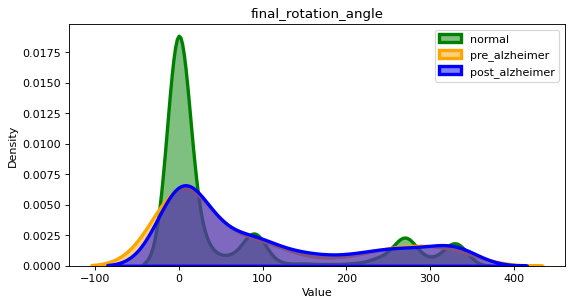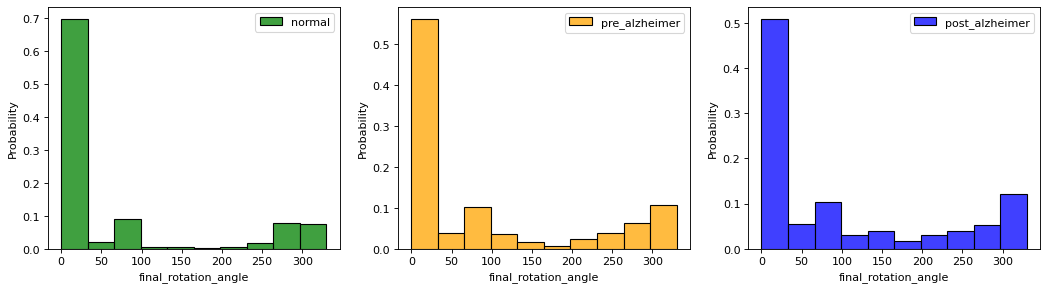### Number of Digits¶

In [ ]:
feature = 'number_of_digits'
feature_describe(feature)
show_distribution(feature)
show_distribution_hist(feature)
clock_features.append(feature)
regr_features.append(feature)

Data type: float64
Number of missing values: 0.23%
Correlation with the diagnosis: -0.21
Min: 1.0
Mean: 10.3
Max: 17.0
Number of unique values: 17
Example of unique values: 12.0, 7.0, 2.0, 11.0, 4.0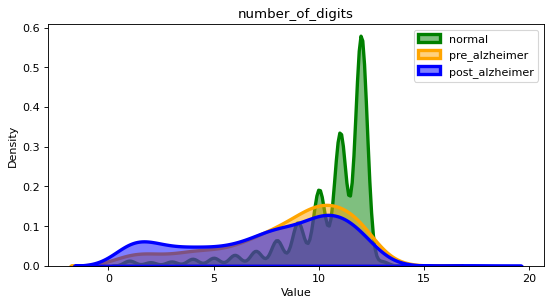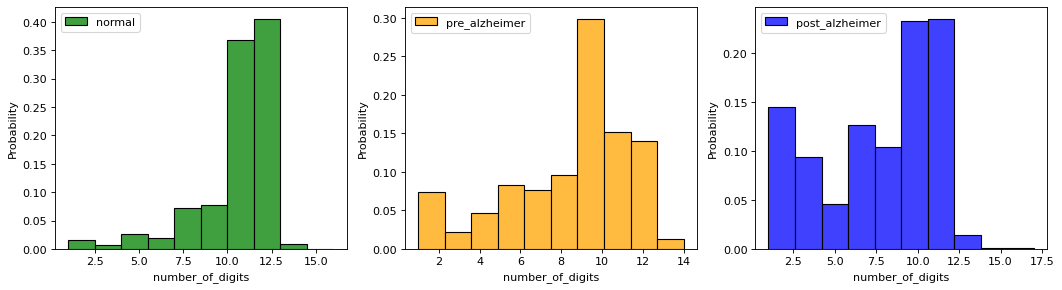### Missing Digit Dummy Variables¶

In [ ]:
feature = 'missing_digit_1'
# similary we have 11 other variables for all the other digits (missing_digit_2, missing_digit_3, etc.)

feature_describe(feature)
show_distribution(feature)
show_distribution_hist(feature)

for i in range(1, 13):
feature = 'missing_digit_{}'.format(i)
cat_features.append(feature)
clock_features.append(feature)

Data type: float64
Number of missing values: 0.23%
Correlation with the diagnosis: 0.12
Min: 0.0
Mean: 0.22
Max: 1.0
Number of unique values: 2
Example of unique values: 0.0, 1.0, nan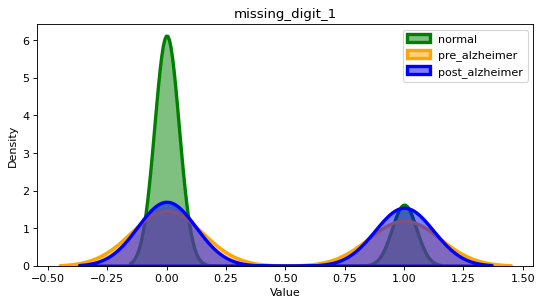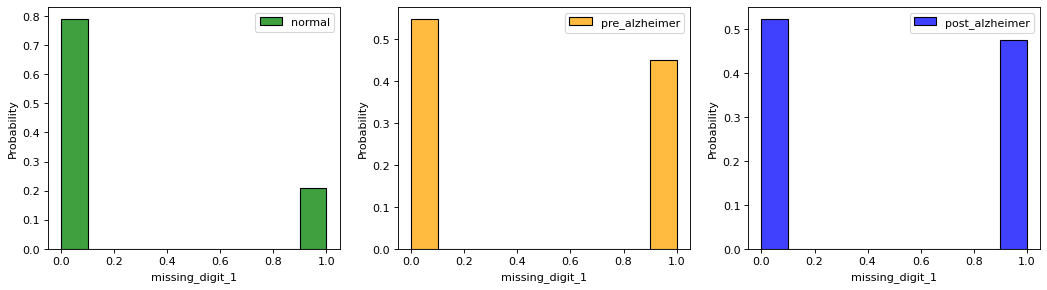### Deviation of Axis Digits (3, 6, 9 and 12) from Mid Axes¶

In [ ]:
feature = 'deviation_dist_from_mid_axis'
feature_describe(feature)
show_distribution(feature)
show_distribution_hist(feature)
clock_features.append(feature)
regr_features.append(feature)

Data type: float64
Number of missing values: 1.77%
Correlation with the diagnosis: 0.04
Min: 0.0
Mean: 32.2
Max: 125.71
Number of unique values: 4336
Example of unique values: 14.1, 21.12, 19.66, 34.02, 8.71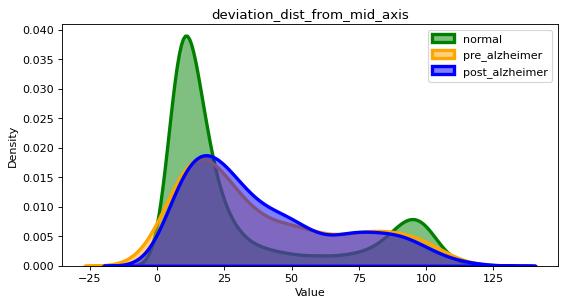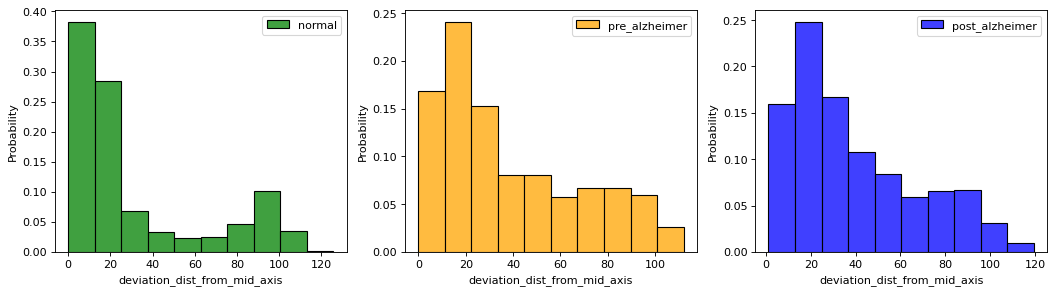### Between Axis Digits Angle Metrics¶

In [ ]:
feature = 'between_axis_digits_angle_sum'
feature_describe(feature)
show_distribution(feature)
show_distribution_hist(feature)
clock_features.append(feature)
regr_features.append(feature)

Data type: float64
Number of missing values: 11.09%
Correlation with the diagnosis: -0.08
Min: 0.0
Mean: 352.14
Max: 360.0
Number of unique values: 74
Example of unique values: nan, 360.0, 0.0, 352.32, 352.7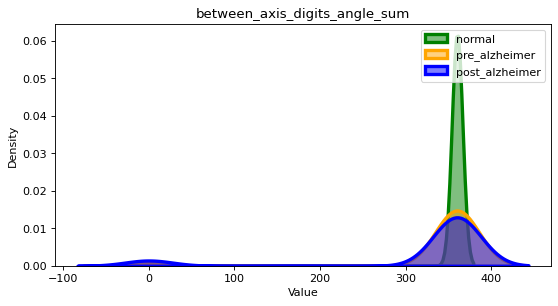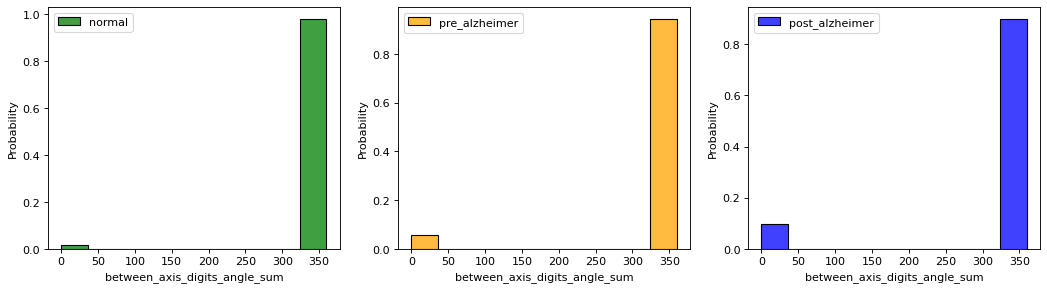In [ ]:
feature = 'between_axis_digits_angle_var'
feature_describe(feature)
show_distribution(feature)
show_distribution_hist(feature)
clock_features.append(feature)
regr_features.append(feature)

Data type: float64
Number of missing values: 5.82%
Correlation with the diagnosis: 0.12
Min: 0.0
Mean: 2587.13
Max: 63116.01
Number of unique values: 30816
Example of unique values: 225.27, 382.13, 439.97, 6465.58, 59.51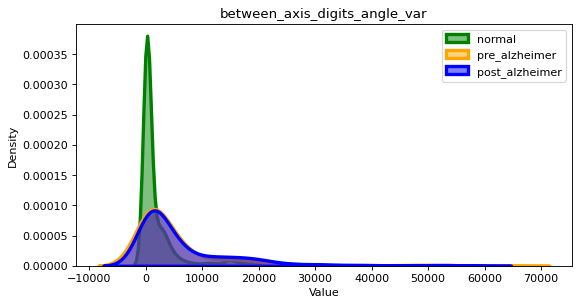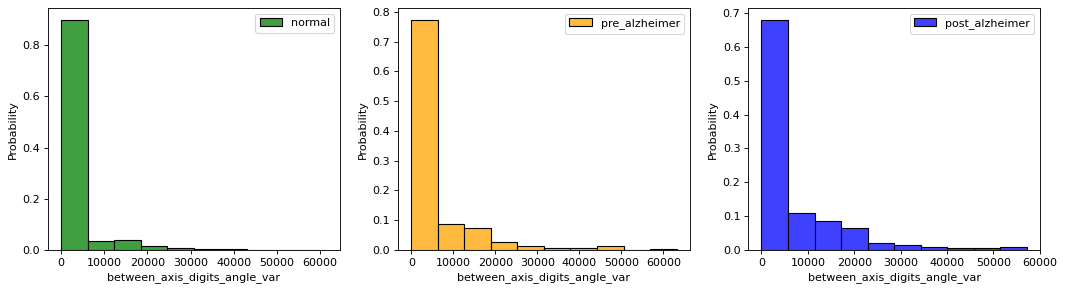### Between Digits Angle Metrics¶

In [ ]:
feature = 'between_digits_angle_cw_sum'
feature_describe(feature)
show_distribution(feature)
show_distribution_hist(feature)
clock_features.append(feature)
regr_features.append(feature)

Data type: float64
Number of missing values: 38.9%
Correlation with the diagnosis: -0.12
Min: 0.0
Mean: 355.1
Max: 360.0
Number of unique values: 4
Example of unique values: 360.0, nan, 0.0, 343.05, 180.0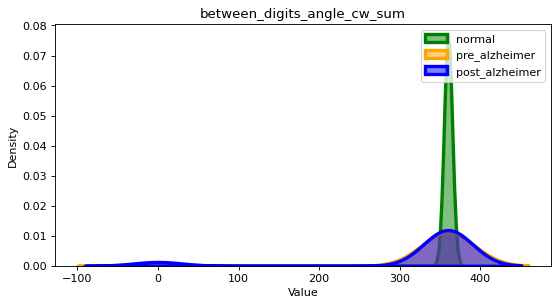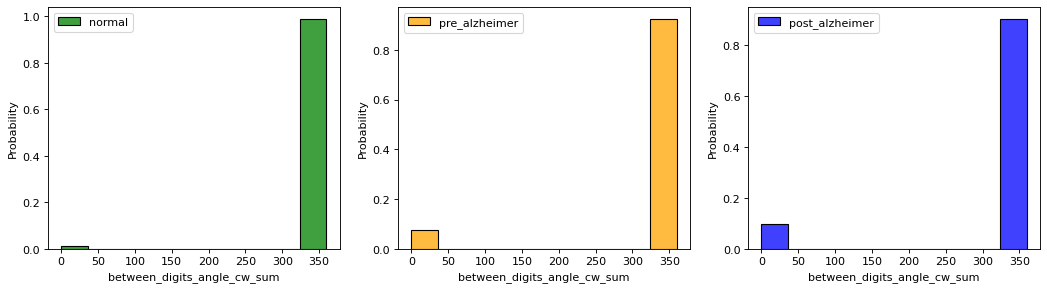In [ ]:
feature = 'between_digits_angle_cw_var'
feature_describe(feature)
show_distribution(feature)
show_distribution_hist(feature)
clock_features.append(feature)
regr_features.append(feature)

Data type: float64
Number of missing values: 2.11%
Correlation with the diagnosis: 0.18
Min: 0.0
Mean: 3081.78
Max: 63259.73
Number of unique values: 32079
Example of unique values: 72.27, 69.82, 49.54, 13467.73, 50.76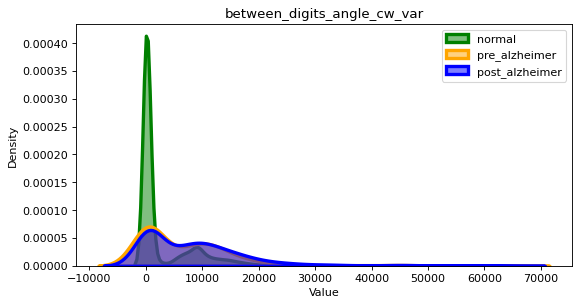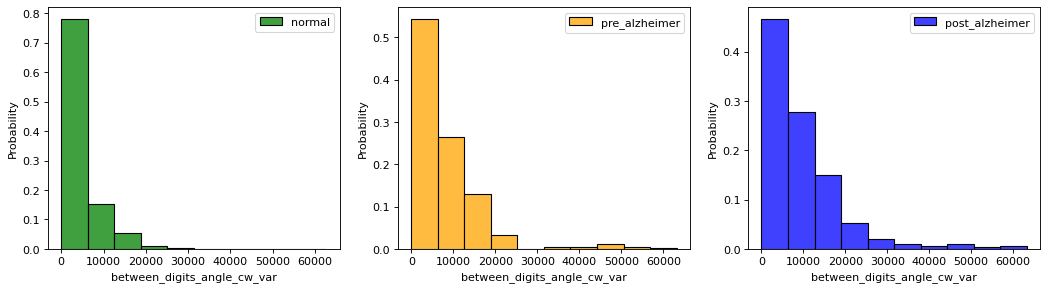In [ ]:
feature = 'between_digits_angle_ccw_sum'
feature_describe(feature)
show_distribution(feature)
show_distribution_hist(feature)
clock_features.append(feature)
regr_features.append(feature)

Data type: float64
Number of missing values: 97.43%
Correlation with the diagnosis: 0.04
Min: 0.0
Mean: 243.83
Max: 360.0
Number of unique values: 3
Example of unique values: nan, 360.0, 0.0, 228.66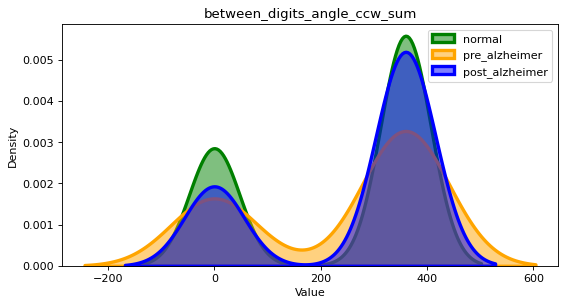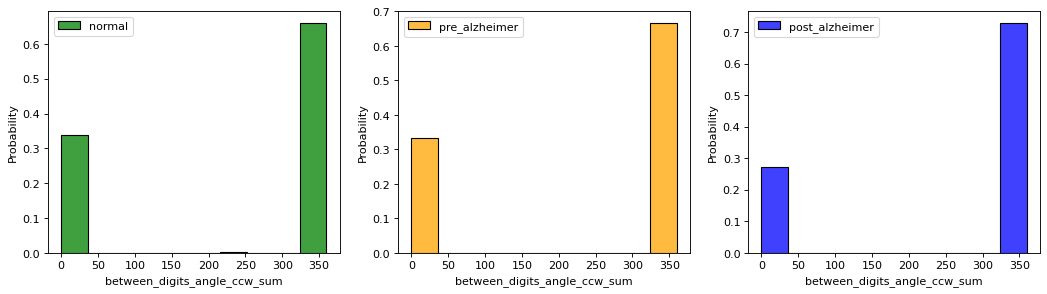In [ ]:
feature = 'between_digits_angle_ccw_var'
feature_describe(feature)
show_distribution(feature)
show_distribution_hist(feature)
clock_features.append(feature)
regr_features.append(feature)

Data type: float64
Number of missing values: 2.11%
Correlation with the diagnosis: 0.17
Min: 0.0
Mean: 3157.42
Max: 63259.73
Number of unique values: 32079
Example of unique values: 72.27, 69.82, 49.54, 13467.73, 50.76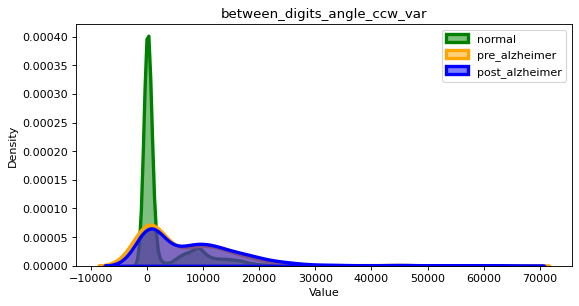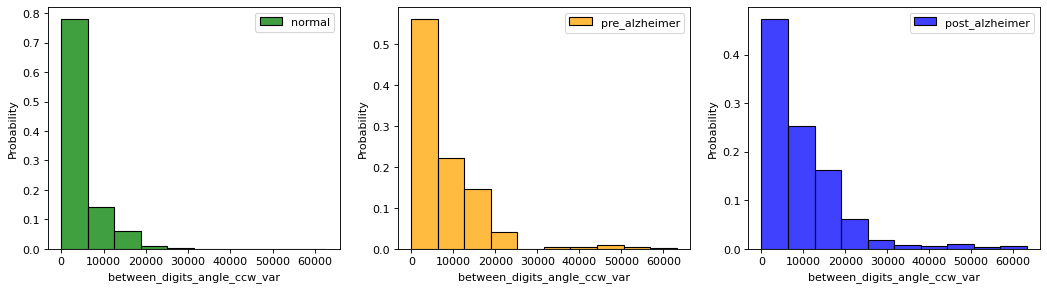### Sequence Flag Clock Wise and Counter Clock Wise¶

In [ ]:
feature = 'sequence_flag_cw'
feature_describe(feature)
show_distribution(feature)
show_distribution_hist(feature)
clock_features.append(feature)
cat_features.append(feature)

Data type: float64
Number of missing values: 0.92%
Correlation with the diagnosis: -0.12
Min: 0.0
Mean: 0.75
Max: 1.0
Number of unique values: 2
Example of unique values: 1.0, 0.0, nan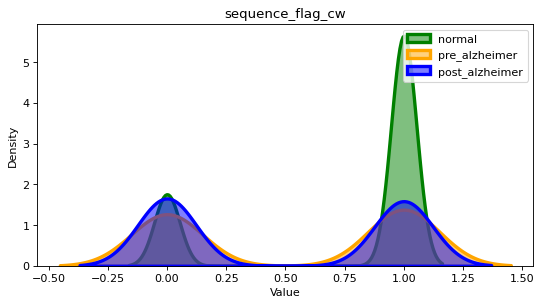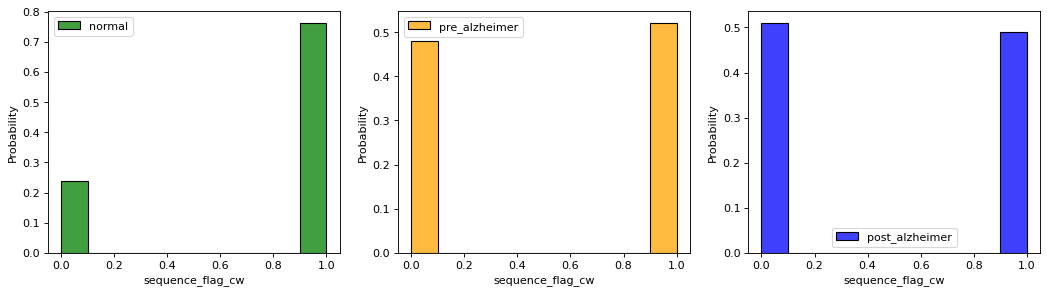In [ ]:
feature = 'sequence_flag_ccw'
feature_describe(feature)
show_distribution(feature)
show_distribution_hist(feature)
clock_features.append(feature)
cat_features.append(feature)

Data type: float64
Number of missing values: 0.92%
Correlation with the diagnosis: 0.14
Min: 0.0
Mean: 0.02
Max: 1.0
Number of unique values: 2
Example of unique values: 0.0, 1.0, nan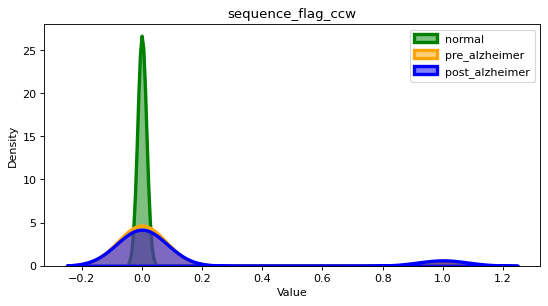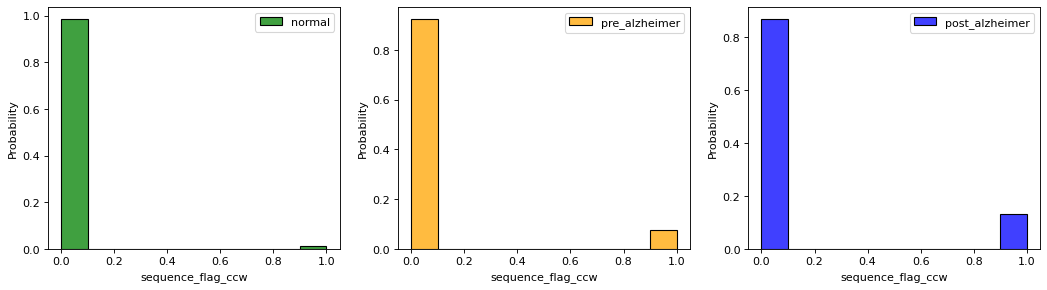### Correlation of clock and digits features¶

In [ ]:
show_corr(clock_features)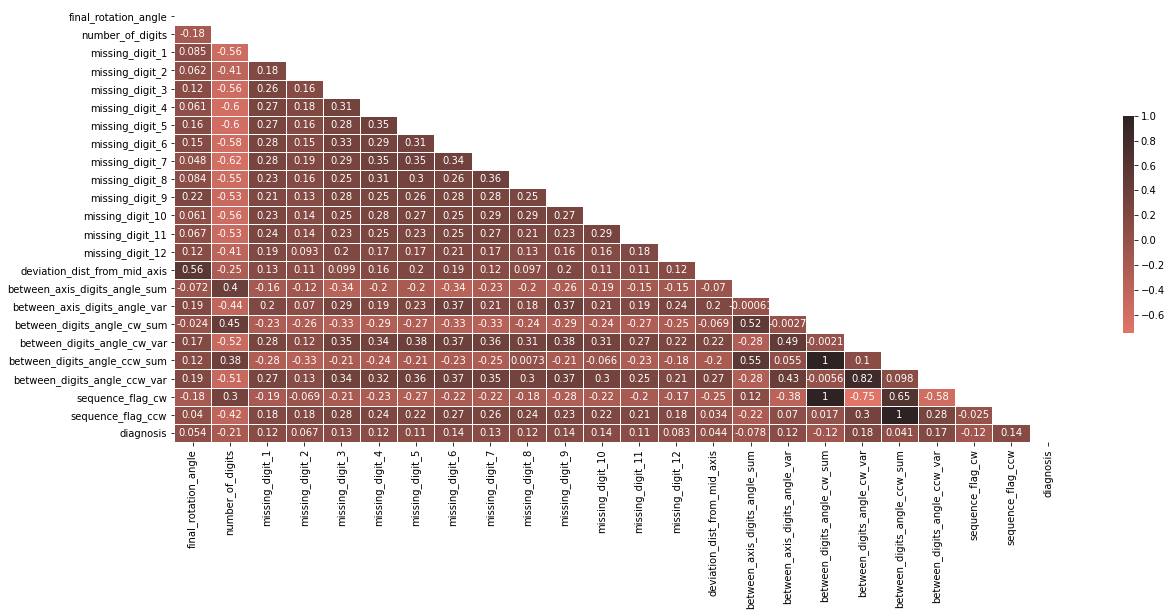## Hand Features¶

In [ ]:
hand_features = []


### Number of Hands¶

In [ ]:
feature = 'number_of_hands'
feature_describe(feature)
show_distribution(feature)
show_distribution_hist(feature)
hand_features.append(feature)
regr_features.append(feature)

Data type: float64
Number of missing values: 6.57%
Correlation with the diagnosis: -0.12
Min: 1.0
Mean: 1.77
Max: 8.0
Number of unique values: 7
Example of unique values: 2.0, 1.0, 4.0, nan, 3.0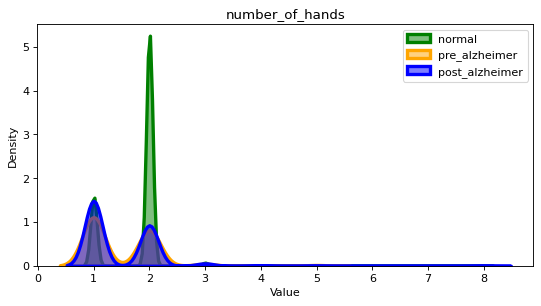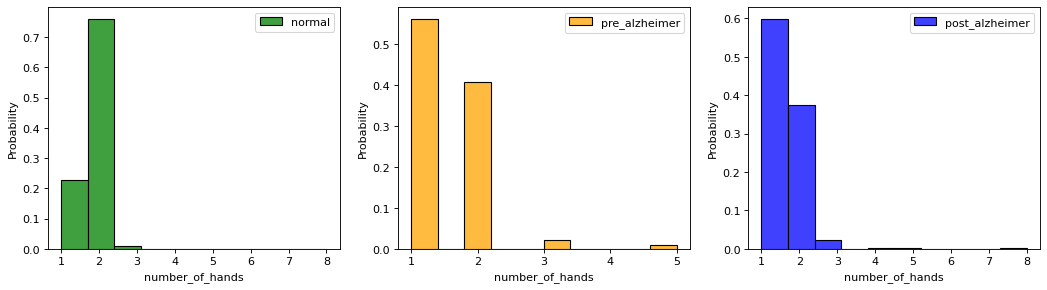In [ ]:
feature = 'hand_count_dummy'
feature_describe(feature)
show_distribution(feature)
show_distribution_hist(feature)
hand_features.append(feature)
cat_features.append(feature)

Data type: float64
Number of missing values: 6.57%
Correlation with the diagnosis: -0.13
Min: 1.0
Mean: 1.77
Max: 3.0
Number of unique values: 3
Example of unique values: 2.0, 1.0, 3.0, nan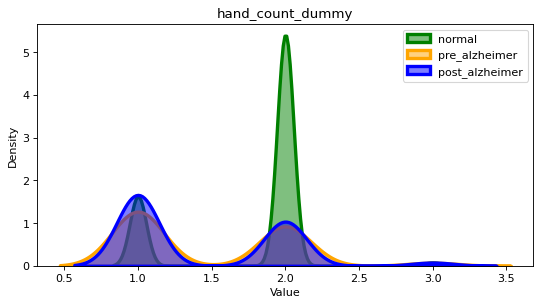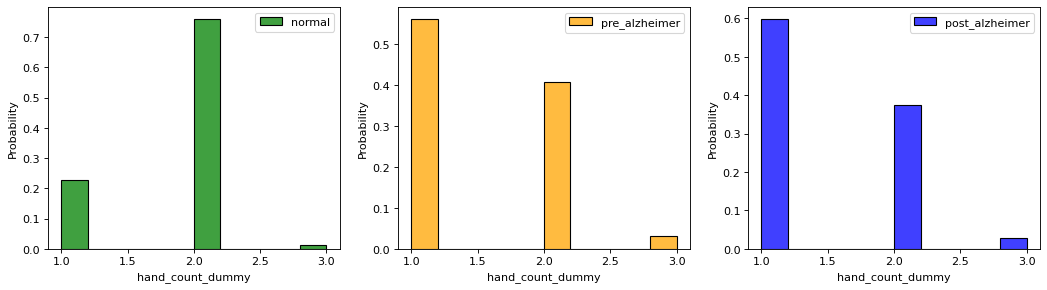### Hand Length¶

In [ ]:
feature = 'hour_hand_length'
feature_describe(feature)
show_distribution(feature)
show_distribution_hist(feature)
hand_features.append(feature)
regr_features.append(feature)

Data type: float64
Number of missing values: 30.25%
Correlation with the diagnosis: -0.0
Min: 23.16
Mean: 60.54
Max: 123.52
Number of unique values: 11931
Example of unique values: 53.16, nan, 70.54, 61.79, 59.54